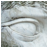SUM UNIVERSAL®Copying is slavery, you have to reach the spirit.

~ Zen Proverb

 All living souls welcome whatever they are ready to cope with; all else they ignore, or pronounce to be monstrous and wrong, or deny to be possible. ~ George Santayana, philosopher (1863-1952)

 You are a child of God. Your playing small does not serve the world. There is nothing enlightening about shrinking, so that other people won’t feel insecure around you. We are born to make manifest the glory of God that is within us. It is not just in some of us; it is in everyone. And as we let our light shine, we unconsciously give other people permission to do the same. As we are liberated from our own fear, our presence automatically liberates others. ~ Marianne Williamson, spiritual activist, lecturer, and author (1952-)

HOME

NUMBERS

NUMBER Pi

PATTERNS

SERVICES

WORKSHOPSReflections ’Job and Numerological Profile’

Numbers don’t lie, and universal laws cannot be corrupted. Everything in the Universe is connected, though we don’t fully comprehend the complexity of the omnipresent cosmic design. No one and nothing can escape it. Whatever we do, is done within a cocoon. What we can do is to accept this and work with the facts to better our conditions within this setup. Often we get a hunch of the underlying facts, but unless it is scientifically proven through logical thinking and by demonstrating it with the help of scientific tools, we block out knowledge that may be true and helpful in our pursuit of advancement. People whose experiences are much more touched by certain facts more likely accept this knowledge. They are the pioneers who help us to create new ways in our pursuit of advancement. Sometimes it requires a leap of faith to follow your intuition. Not everything needs to be explained in detail for it to work.

Right now, I feel the urge to write something about the Inauguration Day of January 20, 2017. As I have stated before, numbers with their specific vibrational energy exert an influence in people’s life who have a specific connection to these numbers. The next President of the United States who will be inaugurated on January 20, 2017 will be on that specific day under the vibrational energy of number 13 and/or 4 (1+20+2017= 2038 and 2+0+3+8=13 and 1+3=4). Number four is his life lesson number, and throughout his life he will be confronted with the metaphysical properties of this number through similar experiences. The day of his inauguration will be in sync with his life lesson number. It’s like two entities shake hands with the understanding of co-operation with each other. As a reminder, the “number four embodies the qualities of building something tangible through a logical structured system and submitting to earthly manifestations in order to construct an orderly and stable foundation. Four is the symbol for continuity and transformation, order and system, form and substance, formation and material manifestation.”

In regards to the office of the presidency, his invigoration and destiny number are in sync with the United States President’s Office. As mentioned earlier in one of my writings, the numerical equivalent of this entity is number five: [President of the United States of America: P=16/7 (1+6=7), R=18/9 (1+8=9), E=5, S=19/1 (1+9=10 and 1+0=1), I=9, D=4, E=5, N=14/5 (1+4=5), T=20/2 (2+0=2), O=15/6 (1+5=6), F=6, T=20/2 (2+0=2), H=8, E=5, U=21/3 (2+1=3), N=14/5 (1+4=5), I=9, T=20/2 (2+0=2), E=5, D=4, S=19/1 (1+9=10 and 1+0=1), T=20/2 (2+0=2), A=1,T=20/2 (2+0=2), E=5, S=19/1 (1+9=10 and 1+0=1), O=15/6 (1+5=6), F=6, A=1, M=13/4 (1+3=4), E=5, R=18/9 (1+8=9), I=9, C=3, A=1 and
7+9+5+1+9+4+5+5+2+6+6+2+8+5+3+5+9+2+5+4+1+2+1+2+5+1+6+6+1+4+5+9+9+3+1=158 and
1+5+8=14 and 1+4=5].
Donald Trump was born on June 14th, therefore his invigoration number, his own individual independence day number, the number of the day he was born, is number five (1+4=5), and his destiny number, derived from his name, is also number five: [Donald John Trump: D=4, O=15/6 (1+5=6), N=14/5 (1+4=5), A=1, L=12/3 (1+2=3), D=4, J=10/1 (1+0=1), O=15/6 (1+5=6), H=8, N=14/5 (1+4=5), T=20/2 (2+0=2), R=18/9 (1+8=9), U=21/3 (2+1=3), M=13/4 (1+3=4), P=16/7 (1+6=7) and
4+6+5+1+3+4+1+6+8+5+2+9+3+4+7=68 and 6+8=14 and 1+4=5].
The vibrational energy of number five manifests in Donald Trump’s numerological profile through his invigoration and destiny number and has a strong effect in his life. The office of the presidency will support this energetic field. As a reminder, the “number five embodies the qualities of being dynamic and intellectual, operating under freedom and independence, and being under the flux of change. Five is the symbol of the human being, it stands for the phenomenal world in its entirety—five senses and the forms of matter open and responsive to sense-perception, it is the symbol for the microcosm.” We will see how all this will work out. It’s a very interesting and challenging setup.

© Jan 20, 2017 Ute Sonja Medley (SUM UNIVERSAL)

We live not only our own lives but, whether we know it or not, also the life of our time.

~ Laurens van der Post